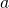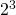# Why is anything the power of 0 always 1?Now I know this is one known fact that students just accept. Why is it that anything raised to the power of 0 is 1? We know that, meaning we take the 2 and multiply it three times. So wouldn’tmean we multiply 2 zeroth times, and get back 2? So thats baffling.

This can be solved intuitively, like how we looked atpreviously. We can look atas the number of ways to arrange a set ofnumber with numbers fromtonumbers.

Consider. This means we arrange the set of 3 numbers where the numbers are just 1 and 2. So we haveAnd we find the number of ways to be eight!

•Aravindan Sai Narayanan

0^0 is undefined.It doesn’t make sense to say that 0^0 is always one.To overcome this problem when a function of the form f(x)^g(x) has f(x) approaching zero as x tends to a and thus,f(x) approaches zero and when x tends to a given point a ,g(x) approaches zero.Thus,as x tends to a ,we have f(x)^g(x) as 0^0.0^0 can be any value and to overcome this indeterminate form, we need to first transform it to 0*infinity form; followed by changing it to infinity/infinity or 0/0 form;afterwhich,we apply L Hospital’s Rule to evaluate the limit as x tends to a.Other indeterminate forms include infinity^0, 0^infinity,1^infinity ,0*infinity,infinity-infinity, infinity/infinity and 0/0.See the attached link for more and detailed information about indeterminate forms /Users/akshayleninsingapore/Downloads/Indeterminate_form.pdf

Not readable? Change text.